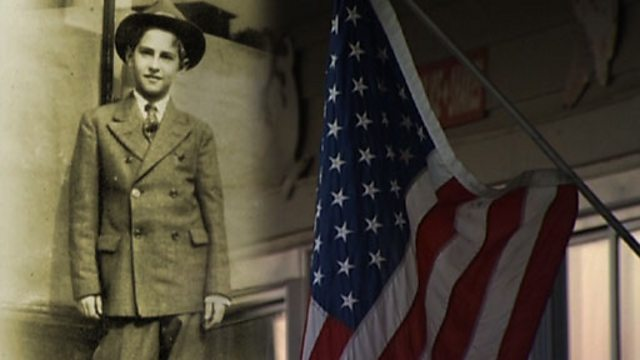# The continuum hypothesis

Marcus du Sautoy looks at the work Paul Cohen did in trying to prove the continuum hypothesis, which asked whether there was a set of numbers between the whole numbers and the infinite decimals. Cohen ended up with two worlds, one where the hypothesis was true, and one where it wasn't, both with consistent mathematics. Godel checked his proof and found it to be correct - leaving the mathematical world a major problem.

3 minutes